• 国外大牛编写的程序，形象生动地说明了图像在频域内的分解与合成
具体实现源码和程序：https://download.csdn.net/download/qq_43145360/12626442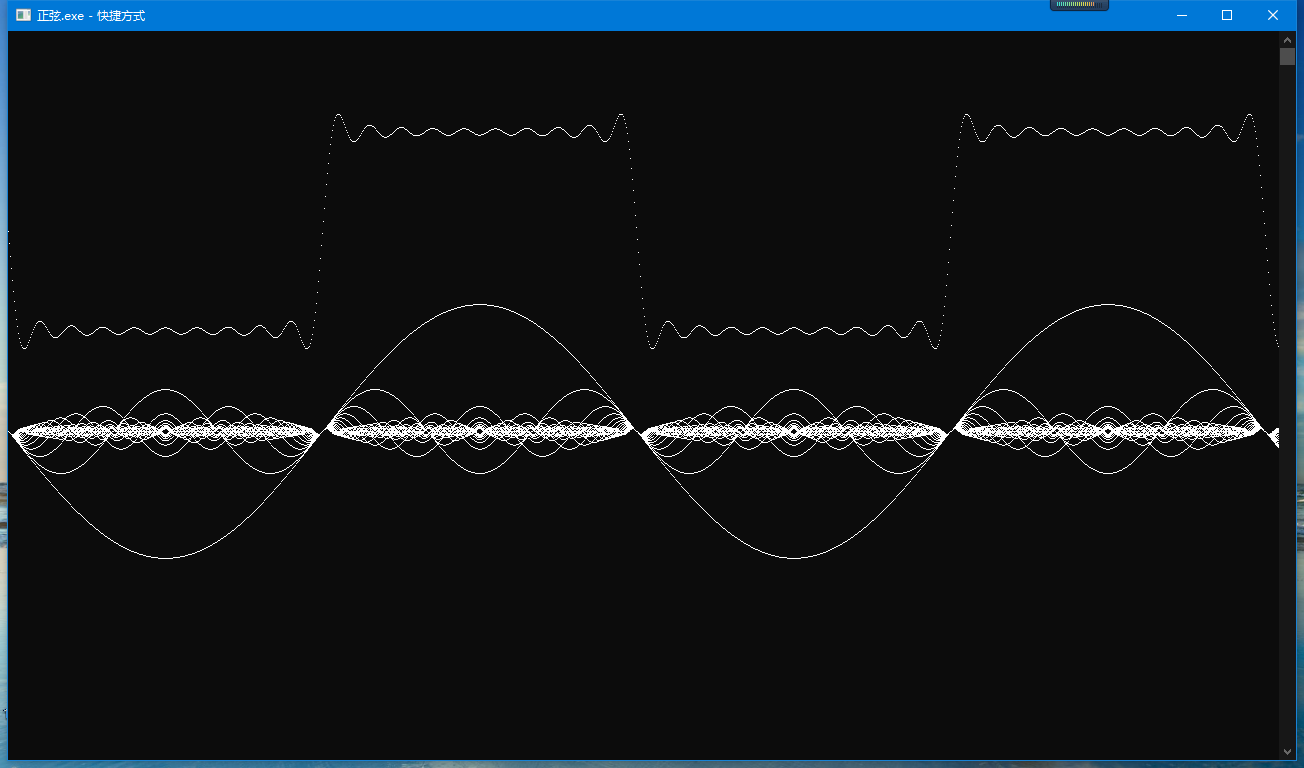展开全文c++
• 计 算 机 现 代 化 2011 年第 9 期 JISUANJI YU XIANDAIHUA 总第 193 期 文章编号:1006-2475( 2011) 09-0156-03 收稿日期:2011-06-29 基金项目: 咸阳师范学院校级项目( 200802005) ; 咸阳师范学院校级精品课程...
计 算 机 与 现 代 化 2011 年第 9 期 JISUANJI YU XIANDAIHUA 总第 193 期 文章编号:1006-2475( 2011) 09-0156-03 收稿日期:2011-06-29 基金项目: 咸阳师范学院校级项目( 200802005) ; 咸阳师范学院校级精品课程项目( 200812006) 作者简介: 郗艳华( 1974-)，女，陕西蒲城人，咸阳师范学院物理与电子工程学院讲师，硕士，研究方向: 信号处理与电子技术。 基于 Matlab 周期信号的分解与合成 郗艳华 ( 咸阳师范学院物理与电子工程学院，陕西 咸阳 712000) 摘要: 分析三角形式傅里叶级数中周期信号的分解与合成，用 Matlab 软件对方波信号的分解与合成进行仿真。结果显示方波信号可以分解成直流分量和不同频率余弦分量( 或正弦分量) 的叠加; 满足一定关系的直流信号和有限次谐波分量之和可以近似表示方波信号，对方波信号合成过程中误差和吉布斯现象进行分析。利用 Matlab 软件仿真可以直观地理解周期信号的分解与合成原理，它对于建立信号频谱的概念以及系统频谱分析都有非常重要作用。 关键词: 周期信号; 分解与合成; 吉布斯现象 中图分类号: TN702 文献标识码: A doi: 10． 3969/j． issn． 1006-2475． 2011． 09． 042 Periodic Signal’s Decomposition and Composition Based on Matlab XI Yan-hua ( School of Physics and Electronics Engineering，Xianyang Normal University，Xianyang 712000，China) Abstract: Decomposition and composition of periodic signal is analyzed with trigonometric form of Fourier series in signal and lin-ear systems，and square wave’s decomposition and composition is simulated with Matlab． The experiment result shows that the square wave can be decomposed into the summation of DC component and different frequency cosine components ( or sine compo-nents) ． The sum of the DC signal and limited harmonic components under certain conditions can be approximated the square wave． The error and Gibbs phenomenon are analyzed in the process of square wave’s composition． The principle of periodic sig-nal’s decomposition and composition can be comprehended with software simulation，it is very important to under
展开全文• 文章目录一、信号的合成分解（一）傅里叶级数（二）将正弦波合成方波二、信号的相关性及傅里叶系数的计算（一）信号的相关性（二）傅里叶系数（三）相关性的验证及幅值、相位的计算三、信号的分解从信号合成到信号...


数字信号处理系列博客：
数字信号处理之信号的合成、分解、相关性及傅里叶系数的计算
数字信号处理之从傅里叶系数到DFT

文章目录一、信号的合成和分解（一）傅里叶级数（二）将正弦波合成方波二、信号的相关性及傅里叶系数的计算（一）信号的相关性（二）傅里叶系数（三）相关性的验证及幅值、相位的计算三、信号的分解从信号合成到信号分解最后
一、信号的合成和分解

任何信号都可以由正弦波合成，反之，任何信号都可以分解为正弦波的叠加，这是傅里叶变换告诉我们的。

（一）傅里叶级数

由公式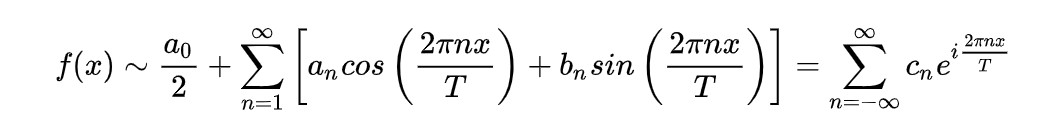可以知道一个信号可以由正弦波叠加而成

（二）将正弦波合成方波

使用matlab生成基波及3、5、7、9、11次谐波

w=1000;%基波频率，单位Hz
f1=sin(2*pi*w*t);%基波信号
f3=sin(2*pi*3*w*t)/3;%3次谐波信号
f5=sin(2*pi*5*w*t)/5;%5次谐波信号
f7=sin(2*pi*7*w*t)/7;%7次谐波信号
f9=sin(2*pi*9*w*t)/9;%9次谐波信号
f11=sin(2*pi*11*w*t)/11;%11次谐波信号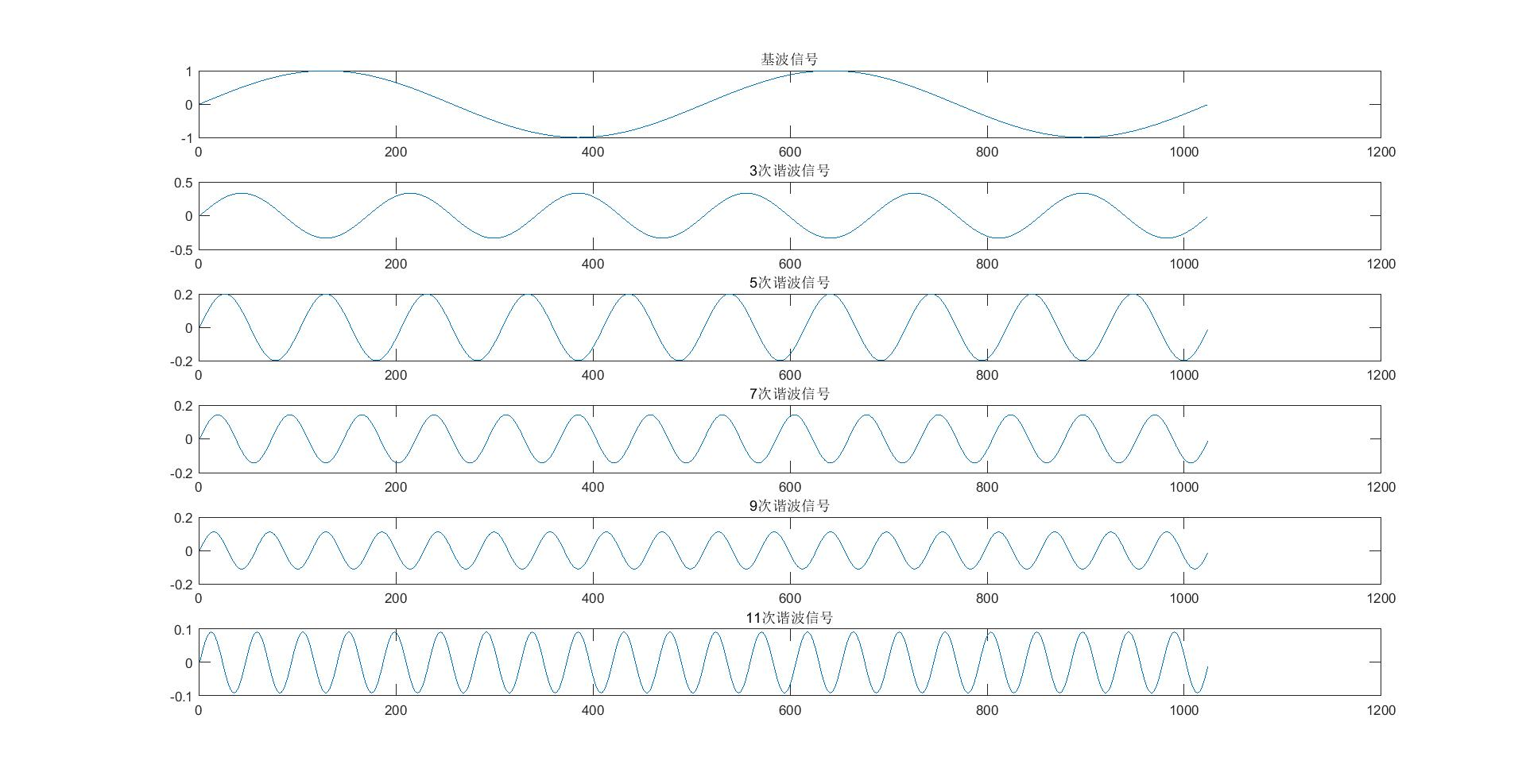将基波及3、5、7、9、11次谐波进行叠加

f=f1+f3+f5+f7+f9+f11;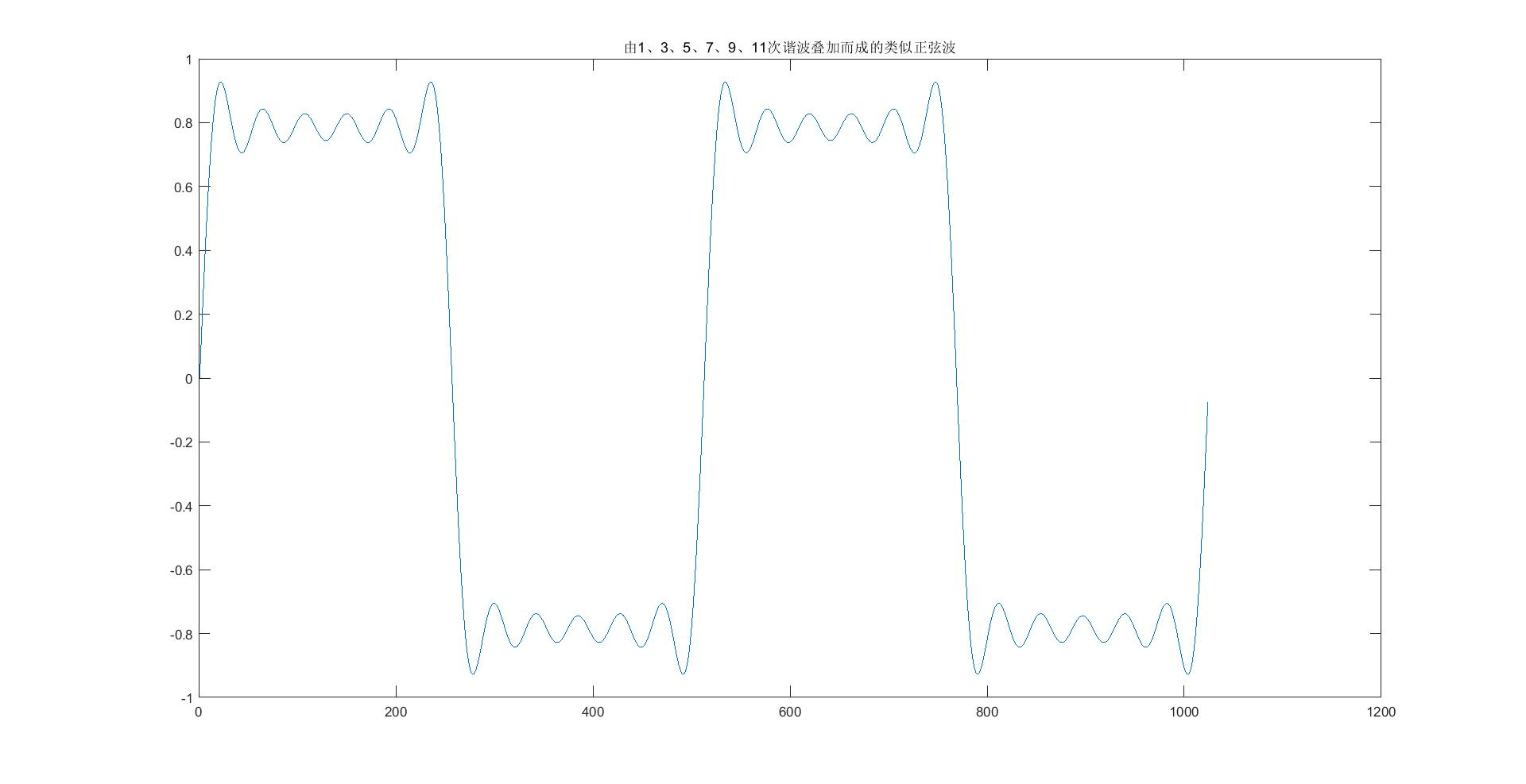这里只是使用了基波及3、5、7、9、11次谐波来叠加，已经可以看得出方波的雏形了，如果使用更多的13、15、17、19。。。次谐波来叠加则会更向方波，事实上一个完整的方波是无限多、无限高次谐波的叠加。另外提一点，即使使用无限多、无限高次谐波来合成方波仍会发现会在间断点出现剑锋，大概是9%的偏差，这个现象叫做吉伯斯现象，因为当谐波次数取得很大时，间断点尖峰下的面积趋近于零，在均方的意义上合成波形与原方波真值没有区别。
回到顶部

二、信号的相关性及傅里叶系数的计算

（一）信号的相关性

所谓信号的相关性，就是一个信号与一个固定频率信号相乘，如果该信号含有固定频率信号的频率成分，那么乘积的结果作积分不为零，反之为零。因为随机信号不含有固定的频率，所以一个随机信号与任意一个固定频率信号相乘做积分结果为零，这正是傅里叶变换能消除随机干扰的原因

（二）傅里叶系数

由傅里叶系数计算公式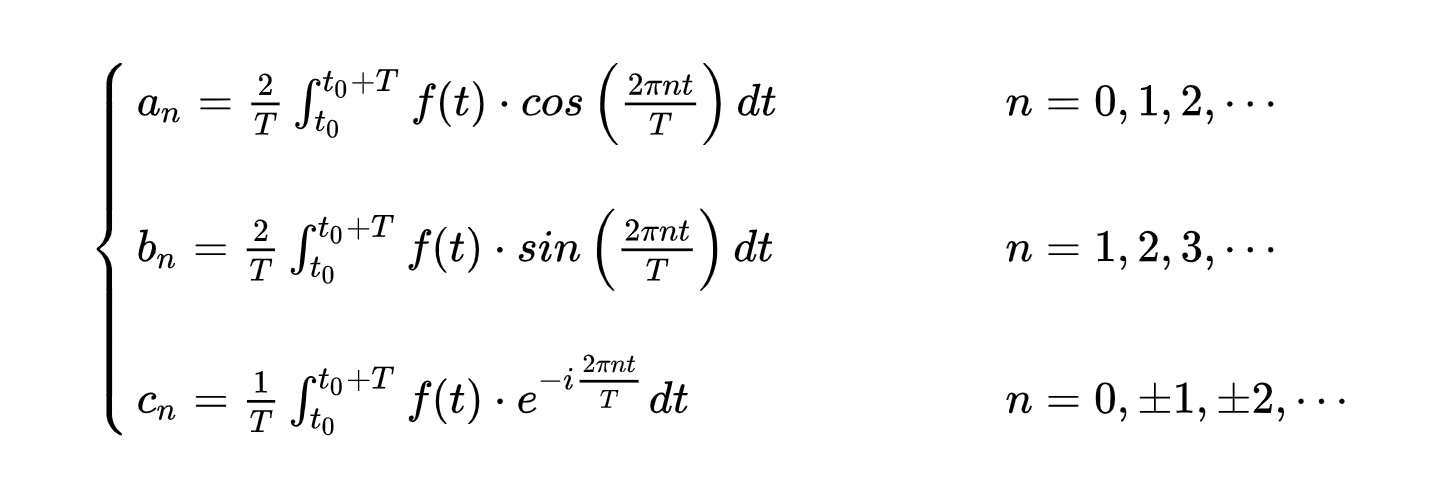可知要计算傅里叶系数就是将原信号与一个固定频率的信号进行相乘然后求积分，我们知道任何一个信号都是由正弦波叠加而成的，我们只要将一个信号与一个已知频率的信号相乘求积分，就知道了在该信号中这个频率的分量，这个过程其实就是求傅里叶系数
回到顶部

（三）相关性的验证及幅值、相位的计算

使用matlab生成相关函数，该相关函数的频率与合成信号的三次谐波频率相同

f_cos=cos(2*pi*3*w*t);%相关函数，用于与合成信号作相关
f_sin=sin(2*pi*3*w*t);%相关函数，用于与合成信号作相关


这里提一点，为什么要用两个信号（cos、sin）来乘呢？你可以把这个过程当做信号的分解，其实这两个信号（cos、sin）是两个正交信号，相当于把原信号分解到两个正交信号上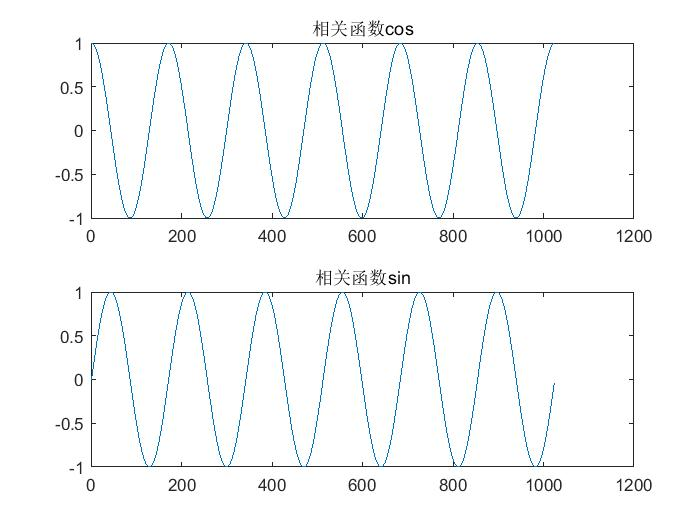将相关信号与合成信号相乘

f_relaven_cos=f.*f_cos;%将合成信号与相关函数相乘，得到实部
f_relaven_sin=f.*f_sin;%将合成信号与相关函数相乘，得到虚部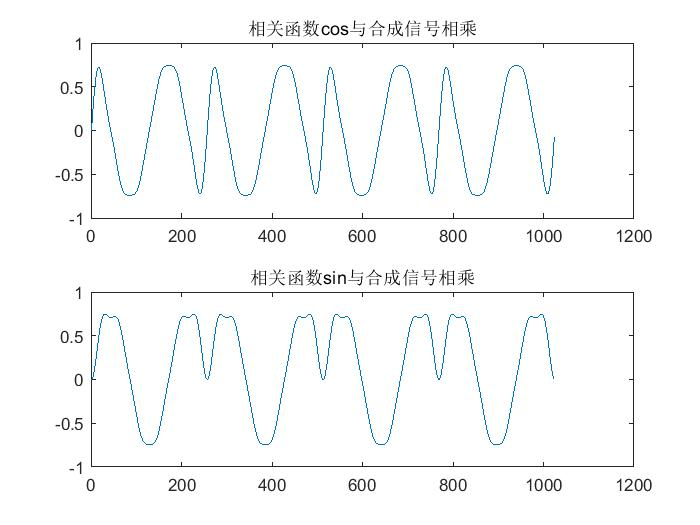将相乘后的信号求积分

sum_data_cos=sum_len(f_relaven_cos,N);%实部求和
sum_data_cos=sum_data_cos/N;
sum_data_sin=sum_len(f_relaven_sin,N);%虚部求和
sum_data_sin=sum_data_sin/N;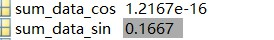求幅值，我们的三次谐波中的幅值正好是一除以三再除以二也就是1.66666666666666…，得出结论原信号与该频率的信号相关，并且原信号中该频率成分分量的幅值为1.666666666666666，

sum_data=sqrt(sum_data_cos^2+sum_data_sin^2);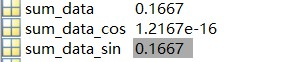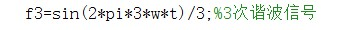若要从能量的角度解释，就是原信号中该频率分量的成分所占能量为0.0278

sum_data_energy=sum_data_cos^2+sum_data_sin^2;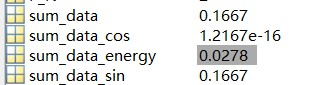再来求一下相角

angle_data=atan(sum_data_sin/sum_data_cos)*180/pi;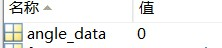初始相位是零看不出结果，我们将三次谐波进行移相，再求相角，注意这时候可能合成的已经可能不是正弦波了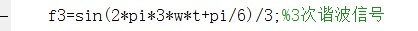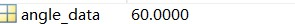验证完毕
回到顶部

三、信号的分解

从信号合成到信号分解

前面的验证我们知道任何一个信号都可以由正弦波合成，那么反过来，任何信号都可以分解为正弦波的叠加。那么问题来了，怎样分解呢？答案就是傅里叶变换。但是傅里叶变换太复杂了，有没有更简单的理解方式呢？我们可以从傅里叶级数出发。
我们知道，欲求一个信号中某种频率的分量，只要将这个信号与欲求频率的信号相乘求积分就能得到这个信号中该频率的含量
再反过来，欲知一个信号中含有那些频率成分及各成分的分量，那就将这个信号与任意频率的信号相乘求积分（作相关），就能得到该信号中所含的所有频率成分及各个分量大小。
但是，与任意频率作相关，这个计算量也太大了吧。。。所以只需要对那奎斯特采样频率一半的信号作相关就够了，并且将任意频率抽取，即每隔多少频率求一次相关，这就是频谱分辨率，怎么越来越像傅里叶变换，没错这就是傅里叶变换。但是傅里叶变换是连续的，在数字系统中无法使用，所以要用离散傅里叶变换，但是离散傅里叶变换计算量还是很大，于是根据离散傅里叶变换的奇、偶、虚、实特特性，快速傅里叶变换诞生了，并且加速了数字信号处理的发展。

最后

本文从信号的合成、分解、相关及傅里叶级数的计算，一非常浅显的方式对信号进行分析，最后引出傅里叶变换、离散傅里叶变换和快速傅里叶变换，之后的内容便是对傅里叶变换、离散傅里叶变换和快速傅里叶变换进行探讨和实现，欲知后事如何请待下回分解。

声明：本文仅作为个人技术交流，所述如有不当之处，欢迎读者批评指正！
转载请注明出处：https://blog.csdn.net/qq_39432978/article/details/89391212

回到顶部


展开全文• 基于Lab view的周期信号分解与合成实验研究利用 LabV IEW 软件可以很方便的将周期信号进行分解, 直观而形象的对周期信号的构成进行研究, 利用傅里叶级数中的有限次正弦谐波近似的合成周期信号。可以看到谐波次数越多...Labview
• 信号系统的仪器实验> 傅立叶分解合成仪说明书 傅立叶分解合成仪说明书
• 【信号系统】信号的正交分解与傅里叶级数

Author：AXYZdong
自动化专业 工科男
有一点思考，有一点想法，有一点理性！

文章目录前言一、信号的正交分解1、信号正交2、正交函数集3、完备正交函数集4、信号的正交分解4.1正交函数的线性组合4.2广义傅里叶级数4.3典型完备正交函数集二、傅里叶级数1、三角形式2、波形的对称性与谐波特性3、指数形式4、周期信号的功率——Parseval等式总结
前言
连续系统的频域分析相关内容。
一、信号的正交分解
1、信号正交
【定义】在 $(t_1,t_2)$ 区间的两个函数 $\varphi_1(t)$ 和 $\varphi_2(t)$ ，若满足$\int_{t_1}^{t_2}\varphi_1(t)\varphi_2^*(t)dt=0$（两个函数的内积为0）
则称 $\varphi_1(t)$ 和 $\varphi_2(t)$ 在 $(t_1,t_2)$ 区间内正交。
说明：实函数正交 $\int_{t_1}^{t_2}\varphi_1(t) \varphi_2(t)dt=0$ （内积为0）
2、正交函数集
若 $n$ 个函数 $\varphi_1(t), \varphi_2(t),..., \varphi_n(t)$ 构成一个函数集，当这些函数在区间 $(t_1,t_2)$ 内满足
$\int_{t_1}^{t_2}\varphi_i(t)\varphi_j^*(t)dt= \begin{cases} 0, i \neq j \\ \\ K_i \neq 0 , i=j \end{cases}$
则称此函数集为在 $(t_1,t_2)$ 区间上的正交函数集。
3、完备正交函数集
如果在正交函数集 $\lbrace \varphi_1(t), \varphi_2(t), ... , \varphi_n(t)\rbrace$ 之外，不存在任何函数 $\varphi(t)$ 满足
$\int_{t_1}^{t_2}\varphi_1(t)\varphi_2^*(t)dt=0 , (i=1,2,...,n)$
则称此函数集为完备正交函数集。
4、信号的正交分解
4.1正交函数的线性组合
设 $n$ 个函数 $\varphi_1(t), \varphi_2(t),..., \varphi_n(t)$ 在区间 $(t_1,t_2)$ 构成一个正交函数空间。将任意函数 $f(t)$ 用这 $n$ 个正交函数的线性组合来近似表示，可表示为：
$f(t) \approx C_1 \varphi_1(t)+ C_2\varphi_2(t)+...+ C_i \varphi_i(t)+...+C_n \varphi_n(t)= \sum_{j=1}^{n}C_j \varphi_j(t)$
说明：存在误差，但是当 $n\to \infty$ 时（完备正交函数集），误差为零。
4.2广义傅里叶级数
任意信号 $f(t)$ 可以表示为无穷多个正交函数之和
$f(t) = C_1 \varphi_1(t)+ C_2\varphi_2(t)+...+ C_i \varphi_i(t)+... = \sum_{i=1}^{n}C_i \varphi_i(t)$
上式称为信号的正交展开式，也称为 广义的傅里叶级数 。

实变函数下：$C_i= \frac{ \int_{t_1}^{t_2}f(t)\varphi_i (t)dt }{\int_{t_1}^{t_2}\varphi_i^2(t) dt}= \frac{1}{K_i} \int_{t_1}^{t_2}f(t)\varphi_i (t)dt$

复变函数下：$C_i= \frac{ \int_{t_1}^{t_2}f(t)\varphi_i ^*(t)dt }{\int_{t_1}^{t_2}\varphi_i(t) \varphi_i^*(t) dt}= \frac{1}{K_i} \int_{t_1}^{t_2}f(t)\varphi_i ^*(t)dt$
$C_i$ 称为 广义傅里叶系数 。
4.3典型完备正交函数集
两组典型的在区间$(t_0,t_0+T)(T=2\pi/ \Omega)$ 上的完备正交函数集
（1）三角函数集 $\lbrace 1, \cos(n\Omega t) ,\sin(n\Omega t) ,n=1,2,...\rbrace$
（2）虚指数函数集 $\lbrace e^{jn\Omega t} ,n=0, \pm 1,\pm 2,...\rbrace$
二、傅里叶级数
$Dirichlet$条件
在一个周期内：（1）如果间断点存在，则间断点的数目应是有限个
（2）极大值和极小值的数目应是有限个
（3）信号满足绝对可积
1、三角形式
直流分量 + $n(n \to \infty)$个正交函数的线性组合。
说明：这里的正交函数属于完备正交函数集（三角函数集）
周期信号 $f(t)$ 其周期为 $T$ 角频率 $\Omega= \frac{2 \pi}{T}$ 当满足 $Dirichlet$ 条件时，它可以分解成如下三角级数：
$f(t)= \frac{a_0}{2} + \sum_{n=1}^{\infty}a_n \cos(n\Omega t) +\sum_{n=1}^{\infty} b_n \sin(n\Omega t)$
$a_n,b_n$ 称为傅里叶系数

$a_n= \frac{2}{T} \int_{-\frac{2}{T}}^{ \frac{2}{T}} f(t) \cos(n\Omega t)dt$

$b_n= \frac{2}{T} \int_{-\frac{2}{T}}^{ \frac{2}{T}} f(t) \sin(n\Omega t)dt$

同频率项合成：$f(t)= \frac{A_0}{2} + \sum_{n=1}^{\infty}A_n \cos(n\Omega t +\varphi_n)$

式中：$A_0=a_0,A_n=\sqrt{a_n^2+b_n^2}, \varphi_n=-\arctan \frac{b_n}{a_n}$

$a_n=A_n\cos \varphi _n , b_n=-A_n\sin \varphi _n,n=1,2,...$

$\frac{A_0}{2}$ ：直流分量；

$A_1 \cos(\Omega t +\varphi _1)$：基波或一次谐波

$A_n \cos(n\Omega t +\varphi _n)$：$n$ 次谐波
2、波形的对称性与谐波特性

对称条件
展开式中所包含成分
$a_n$
$b_n$

偶函数
直流项$+$余弦项
$\frac{T}{4} \int_0^{\frac{2}{T}} f(t) \cos(n\Omega t)dt$
$0$

奇函数
正弦项
0
$\frac{T}{4} \int_0^{\frac{2}{T}} f(t) \sin(n\Omega t)dt$

偶谐函数$f(t)=f(t \pm \frac{T}{2})$
只含偶次谐波
$\frac{T}{4} \int_0^{\frac{2}{T}} f(t) \cos(n\Omega t)dt$
$\frac{T}{4} \int_0^{\frac{2}{T}} f(t) \sin(n\Omega t)dt$

奇谐函数$f(t)=-f(t \pm \frac{T}{2})$
只含奇次谐波
$\frac{T}{4} \int_0^{\frac{2}{T}} f(t) \cos(n\Omega t)dt$
$\frac{T}{4} \int_0^{\frac{2}{T}} f(t) \sin(n\Omega t)dt$

3、指数形式
$f(t)=\sum_{n=-\infty}^{\infty} F_n e^{j n \Omega t}$

复傅里叶系数$F_n= \frac{1}{T} \int_{-\frac{2}{T}}^{ \frac{2}{T}} f(t) e^{-j n \Omega t}dt$
4、周期信号的功率——Parseval等式
平均功率：
$\frac{1}{T} \int_{0}^{T} f^2(t) dt =(\frac{A_0}{2})^2 +\sum_{n=1}^{\infty} \frac{1}{2} A_n^2 =\sum _{n=-\infty}^{\infty} |F_n| ^ 2$
总结
中学学过 矢量的正交分解 ，类比，信号也可以正交分解，任意信号 $f(t)$ 可以表示为无穷多个正交函数之和，完备的 正交函数集 为 傅里叶级数 埋下了伏笔。乍一看傅里叶级数一串儿公式，刚开始可能不理解，如果从信号的正交分解来看，就不难理解了。

「你可能还想看」系列文章：
【信号与系统】笔记合集，你确定不收藏吗？我已经收藏了

笔记记得可能有点粗糙，如有错误之处，还请批评指正 ^ _ ^

如果觉着帮到你的话，点个赞支持一下呢！！！^ _ ^
码字不易，大家的支持就是我坚持下去的动力。点赞后不要忘了关注我哦！


展开全文信号处理
• 众所周知，信号系统讨论的系统是线性时不变系统，而线性时不变系统的特征向量就是e指数函数，所以我们选取特征向量(e指数函数)为基，对时域信号进行分解(即获取它在特征向量基底下的坐标)，这就是傅里叶分析。...
• 软件 Multisim 进行方波信号的产生、分解合成，观察信号波形的分解与合成，加深对信号频谱的理解。信号处理 simulink
• 周期信号的傅里叶级数分解（以方波为例） 1、什么是周期信号？ 周期信号是经过一定时间可以重复的信号，满足条件：x(t)=x(t+nT);n=1,2,3,4,… 其中，T称为信号的周期，其倒数f=1/T为信号的频率。 2、周期信号的三角...狄利克雷条件
• 目录 傅里叶级数变换的理解  1 为什么我们要分解一个函数 ... 分解与合成是一种朴素的思想，有的时候可以将一个复杂的问题分解成若干个小问题逐个击破，当每一个小的问题解决以后，大的问题也就随之...
• 中国科技论文在线 基于 MATLAB GUI 的傅里叶变换分析的仿 真设计 高恒伟宋余君何辉* 中国矿业大学信电学院江苏徐州 221008 摘要本文简要阐述了...周期方波信 号的分解与合成的基本原理并利用MATLAB7.6 的图形用户界
• 傅里叶级数与傅里叶变换的关系应用   摘要：傅里叶级数是对周期性现象做数学上的分析，而傅里叶变换则可以看作傅里叶级数的极限形式，它也可以看作是对周期现象进行数学上的分析。除此之外，傅里叶变换还是...
• 合成孔径雷成像（一）— 傅里叶变换基础1 傅里叶变换是一种非常常见并且实用的数学工具，特别是在分析随机信号，解决先行问题。傅里叶变换可以简单的定义为一个线性算子，目的是将一个域中的函数或者信号映射到...信号处理 算法
• 内容包括小波分析与傅里叶分析的对比，积分小波变换，正交多尺度分析及正交小波，紧支正交小波，小波和小波包的分解合成算法，时间-频率分析及相应算法等内容。是一本比较好的书籍！如果您对小波有兴趣，可以参看...
• 分解与合成是一种朴素的思想，有的时候可以将一个复杂的问题分解成若干个小问题逐个击破，当每一个小的问题解决以后，大的问题也就随之解决。傅里叶变换也是基于这个思想的，它可以将一个函数f(x)分解成若干正弦、...
• 1.傅里叶分析方法的理论基础 2 傅里叶分析方法概述基本框架 3 函数/信号的积分 4函数/信号之间的相关性正交 5. 基本正交信号的选择 6.傅里叶分析的基本思路 7 傅里叶分析的9大步骤
• ## 傅里叶级数

万次阅读 2017-12-23 19:22:56
傅里叶级数到连续傅里叶 这次的内容是有关傅里叶变换的，本文重点在于理解傅里叶变换的思想，对于公式的推导也仅仅是泛泛而谈，如有不严谨之处还望大家莫怪。在正式推导公式之前我想先简单的谈一谈对函数进行...
• 以上内容有参考信号的matlab辅导书，频率周期系数的关系还有一点令人迷惑的地方，下午约了同学一起打羽毛球，三角脉冲信号以后再说吧，大概率会鸽 康康这篇文章，五分钟写出一个可以滑动条拖动的矩形脉冲合成的...信号
•  时域中分解出的每个正弦波对应频域中的一个频率。运用频域的首要条件就是能够将波形从时域变换到频域，用傅立叶变换可以做到这一点。   对于 模拟信号，采用傅立叶积分 。傅里叶积分是在整个时间轴上从 负无穷...
• 【DWT笔记】傅里叶变换小波变换 一、前言  我们经常接触到的信号，正弦信号，余弦信号，甚至是复杂的心电图、脑电图、地震波信号都是时域上的信号，我们也成为原始信号，但是通常情况下，我们在原始信号...
• 认知是一种傅里叶变换吗？为什么不是？为什么是？人类认识事物，生成规则，其实是通过“概念”（知识）和直觉（关系）来的，“概念”（知识）是一个浓缩的信息载体，...
• ## 傅里叶级数及傅里叶变换

万次阅读 多人点赞 2018-06-17 23:59:23
正弦信号：周期信号的傅里叶级数：用正弦信号逼近方波：如下图：船的振荡频率为 ，跷跷板的频率为 ，小女孩的玩具的振荡频率为 ，如果排列合理的话，三者的波形能合成类似于方波的样子，且此频率规律的正弦波越多...
• ## 傅里叶变换推导

万次阅读 2019-01-11 21:18:58
图像傅里叶变换 ...在当时另一个资历比较浅的数学家傅里叶论断：任何周期函数都可以分解为三角函数之和。有意思的是拉格朗日对此持反对意见并指出：带有棱角的周期函数肯定不能由三角函数合成。由于当时朗格朗......